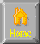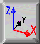```
THEORY OF COMPUTATION

HILBERT - There exists assumptions for all procedures s.t. conclusion
(decision problem)
||
\/
MODEL OF COMPUTATION
1935 ALAN TURING
1. *invents* Idea of Algorithm
2. Turing Machine (TM) if state [A..Z] scanning x then do y & goto
state [A..Z]
3. Universal Turing Machine (UTM)= TM w/ O/S & Tape read. Emulate
Any Algorithm

Turing Machine
Bit->Word->Stream->Program->Output
Formal System
Syntax->Lexicon->Axiom->Heuristic->Theorem
Mathematical
Semantics->Geometry->Analysis->Transform->Tautology

1980 BSS - Blum-Shub-Smale
Flowchart model of computation
space of inputs -> space of outputs ->states -> halting set (Gamma)
||
\/

1936 HALTING PROBLEM
For any turing machine program H , purporting to settle the halting or
non-halting of all turing machine programs there exists program P w/
input I s.t. program H cannot determine if P will halt when processing I

/\
||

GODEL's THEOREM
For any consistent formal system F purporting to settle, prove or disprove.
All statements of arithmetic.  There exists an arithmetical proposition
that can be neither proved nor disproved in this system therefore, the
formal system F is incomplete

1. Godel Numbering : Code scheme translate logical formula
proof into mirror of natural numbers
2. Liar Paradox    : Truth - provability "this sentence is false"
-> "This statement is not provable"
3. Godel Sentence  : "This statement unprovable"
math counterpart = godel sentence
G in all math formalizations
4. Incompleteness  : Prove godel sentence G true but unprovable
if consistent formal system
5. No Escape Clause: New systems w/ added axioms has its own
unprovable godel sentence
6. Consistency     : Math statement A, "Arithmetic is consistent"
prove this is unprovable;  arithmetic as a formal
system too weak to prove own consistency

1950 TURING TEST - Can a machine Think?
Intelligence vs Consciousness
posit only external observed behavior key- eliza

HALTING PROBABILITY
Omega = Prob ( Random program for turing machine will halt)

||
\/
P and NP
1. P Algorithms which run in polynomial time f(problem size) e.g. sort cards)
2. Exponential time algorithms (n!, 10^n, Tower of Hanoi 2^n-1)
3. NP - Nondeterministic Polynomial Time
e.g. routing problem, assignment problem,map coloring prob, bin packing.

```HomeNext ... Optimization